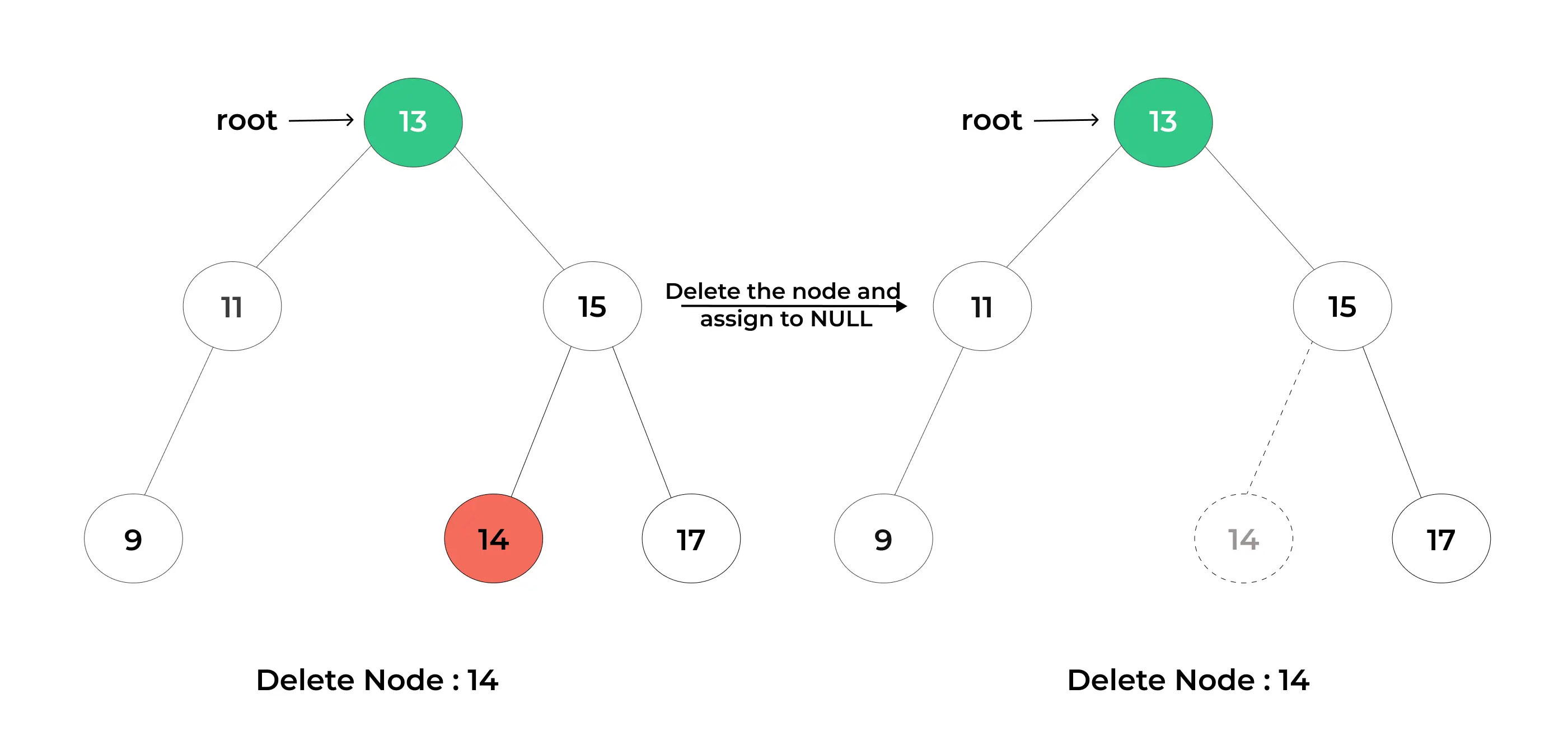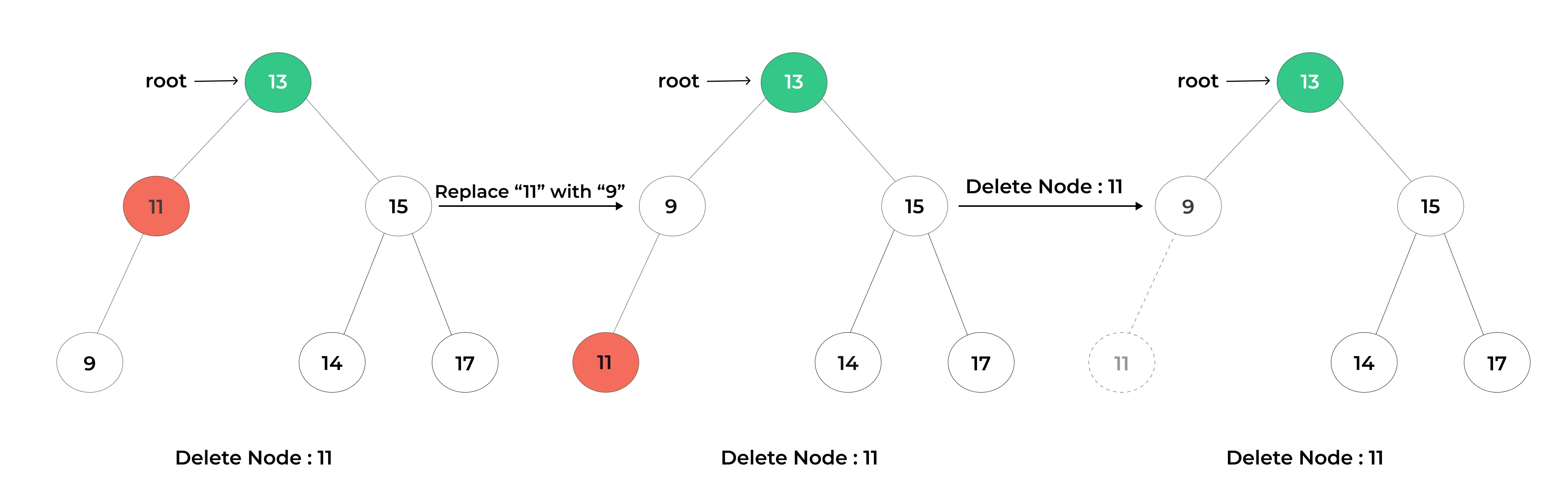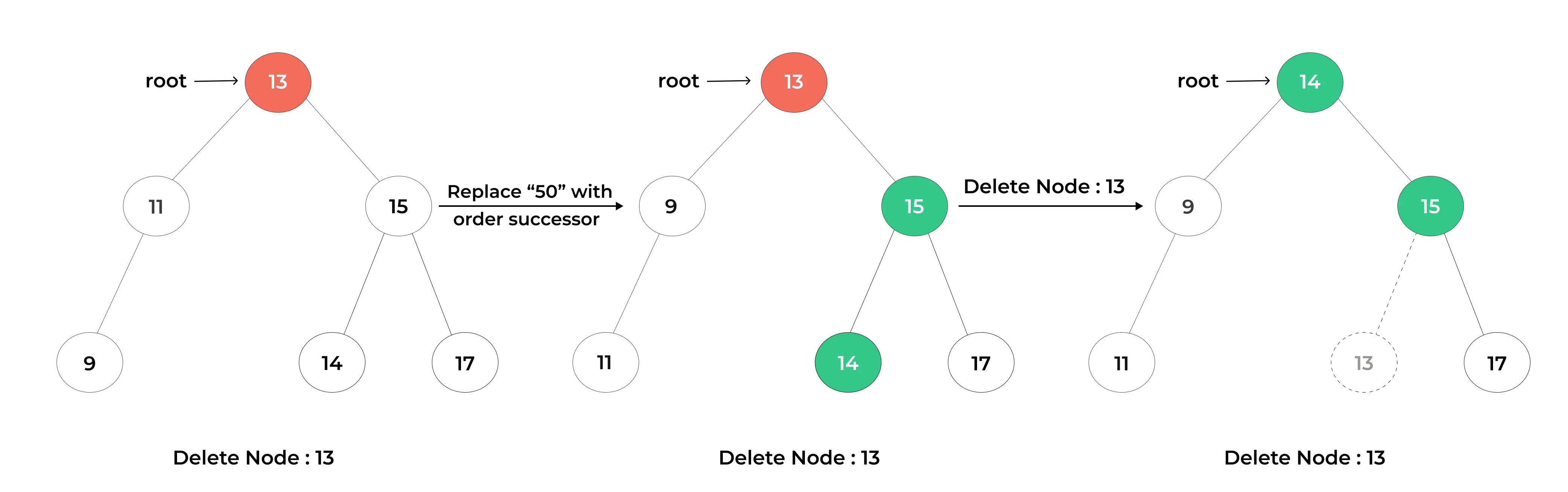# Deletion in Binary Search Tree(BST)## Introduction to Deletion in Binary Search Tree

“Deletion in a Binary Search Tree (BST) is the process of removing a specific node while preserving the tree’s ordering properties.”

In this article, we will explore the fundamentals of Deleting a node, along with this we will learn about scenarios of deletion in Binary Search Tree and then its complexities.

## What is Deletion in Binary Search Tree(BST)?

Deletion refers to the process of removing a specific node from the tree while preserving the ordered structure of the BST. This operation involves handling various scenarios based on the number of children the node has and its position within the tree.

## Deletion Algorithm in a Binary Search Tree (BST):

1. Search for the Node to Delete:

• Start at the root of the BST.
• Compare the key of the node you want to delete with the current node’s key.
• If the key matches the current node’s key, you have found the node to delete.
• If the key is less than the current node’s key, move to the left subtree.
• If the key is greater than the current node’s key, move to the right subtree.
• Repeat this process until you find the node to delete or determine that it does not exist in the tree.

2. Handling Different Deletion Scenarios:

• Once you find the node to delete, consider the following scenarios:

a. Node with No Children (Leaf Node):

• Simply remove the node from the tree by setting its parent’s appropriate child pointer (left or right) to None.

b. Node with One Child:

• If the node has only one child (either left or right), replace the node with its child. Update the parent’s pointer to the child node.

c. Node with Two Children:

• If the node to delete has two children, you need to find its in-order successor (the node with the smallest key in its right subtree) or in-order predecessor (the node with the largest key in its left subtree).
• Copy the in-order successor’s or predecessor’s key to the node you want to delete.
• Then, recursively delete the in-order successor or predecessor node.

3. Updating the Tree:

• After deletion, ensure that the BST’s ordered property is maintained.
• If you replaced a node with a child, the BST remains ordered.
• If you replaced a node with the in-order successor or predecessor, the BST’s order is also preserved.
##### Scenarios of Deletion in Binary Search Tree(BST)

Deletion in a Binary Search Tree (BST) involves various scenarios depending on the number of children a node has and its position within the tree. Let’s explore the different scenarios:

### 1. Node with no Children(Leaf Node):

• In this scenario, the node to be deleted has no children, meaning it’s a leaf node.
• Deletion is straightforward: simply remove the node from the tree.### 2. Node with One Child:

• When the node to be deleted has only one child (either left or right), you can replace the node with its child.
• Update the parent’s pointer to point to the child node.### 3. Node with Two Children:

• Deleting a node with two children is more complex.
• You need to find the node’s in-order successor (the smallest node in its right subtree) or in-order predecessor (the largest node in its left subtree).
• Copy the key of the successor or predecessor to the node to be deleted.
• Then, recursively delete the successor or predecessor node.### Code:

```class TreeNode:
def __init__(self, key):
self.key = key
self.left = None
self.right = None

def inorder_traversal(root):
if root:
inorder_traversal(root.left)
print(root.key, end=" ")
inorder_traversal(root.right)

def find_min(node):
current = node
while current.left:
current = current.left
return current

def delete_node(root, key):
if root is None:
return root

# Search for the node to be deleted
if key < root.key:
root.left = delete_node(root.left, key)
elif key > root.key:
root.right = delete_node(root.right, key)
else:
# Node with only one child or no child
if root.left is None:
return root.right
elif root.right is None:
return root.left

# Node with two children: Get the in-order successor
temp = find_min(root.right)

# Copy the in-order successor's content to this node
root.key = temp.key

# Delete the in-order successor
root.right = delete_node(root.right, temp.key)

return root

if __name__ == "__main__":
root = TreeNode(50)
root.left = TreeNode(30)
root.right = TreeNode(70)
root.left.left = TreeNode(20)
root.left.right = TreeNode(40)
root.right.left = TreeNode(60)
root.right.right = TreeNode(80)

print("Original BST:")
inorder_traversal(root)
print("\n")

key_to_delete = 40
root = delete_node(root, key_to_delete)

print(f"BST after deleting {key_to_delete}:")
inorder_traversal(root)
```

### Output :

```Original BST:
20 30 40 50 60 70 80

BST after deleting 40:
20 30 50 60 70 80
```
##### Explanation
• The provided Python code demonstrates the deletion of a node in a Binary Search Tree (BST). It initializes a BST with different numerical values and then deletes a node with a specified value (40 in this case).
• The delete_node function handles various scenarios for node deletion, such as nodes with zero, one, or two children, ensuring the tree’s ordered structure is preserved.
• After deletion, it prints the BST before and after the operation. In the example output, you can see that the node with the value 40 is successfully deleted, and the BST maintains its ordered structure.

### Code:

```class TreeNode:
def __init__(self, key):
self.key = key
self.left = None
self.right = None

def delete_node(root, key):
if not root:
return root

if key < root.key:
root.left = delete_node(root.left, key)
elif key > root.key:
root.right = delete_node(root.right, key)
else:
if not root.left:
return root.right
elif not root.right:
return root.left

temp = find_min(root.right)
root.key = temp.key
root.right = delete_node(root.right, temp.key)

return root

def find_min(node):
while node.left:
node = node.left
return node

# Example usage:
if __name__ == "__main__":
root = TreeNode(50)
root.left = TreeNode(30)
root.right = TreeNode(70)
root.left.left = TreeNode(20)
root.left.right = TreeNode(40)
root.right.left = TreeNode(60)
root.right.right = TreeNode(80)

key_to_delete = 30
root = delete_node(root, key_to_delete)

print("BST after deleting", key_to_delete, ":")
inorder_traversal(root)```

### Output :

```BST after deleting 30 :
20 40 50 60 70 80
```
##### Explanation
• This optimized Python code deletes a node in a Binary Search Tree (BST) efficiently while preserving the tree’s ordered structure.
• It handles scenarios where a node has zero, one, or two children. The example demonstrates the deletion of the node with the key 30 from the BST and prints the resulting tree.
##### Complexity Analysis for Deletion in Binary Search Tree(BST)

The complexity analysis of deletion in a Binary Search Tree (BST) involves examining both time and space complexities.

Time Complexity:

1. Average-Case Time Complexity: In a balanced BST, the average-case time complexity for deletion is O(log n), where “n” is the number of nodes in the tree. This is because the height of a balanced BST remains logarithmic with respect to the number of nodes.

2. Worst-Case Time Complexity: In the worst-case scenario, when deleting a node with two children (requiring the search for the in-order successor or predecessor), the time complexity can be O(log n). However, the constant factor is larger than in the average case due to the extra steps involved.

3. Worst-Case Scenario: In a highly unbalanced BST (resembling a linked list), the time complexity of deletion can degrade to O(n). This happens when the tree’s height becomes linear, and you may need to traverse the entire height of the tree to find the node to delete.

Space Complexity:

• The space complexity for deletion in a BST is primarily determined by the recursive call stack during the deletion process. In a balanced BST, the space complexity for the recursive call stack is O(log n) on average. This is because the maximum depth of the recursive call stack is limited to the height of the tree.

• In the worst-case scenario, where the tree is highly unbalanced and resembles a linked list, the space complexity can be O(n) in the worst case, as you may need to traverse the entire height of the tree in a linear fashion.

• The space complexity is also influenced by any additional data structures or variables used in the deletion algorithm, but these typically have constant space requirements.

##### Real World Application of Insertion in Binary Search Tree

Deletion in a Binary Search Tree (BST) is a fundamental operation with real-world applications in various domains. Here are some practical scenarios where deletion in BSTs is relevant:

1. Database Management:

• Relational database management systems use BSTs to implement indexes. Deletion is crucial for maintaining data integrity and optimizing database performance by removing outdated or irrelevant data.

2. File Systems:

• File systems use BSTs for directory structures. Deletion of files or directories requires efficient handling to free up storage space and maintain the structure’s organization.

3. Contact Lists and Address Books:

• Applications that manage contact lists or address books often use BSTs to store and search for contacts by name. Deletion allows users to remove or update contact information.
##### To wrap it up:

In conclusion, the process of deletion in a Binary Search Tree (BST) is a fundamental and intricate operation with wide-ranging applications in computer science and real-world scenarios. It involves carefully maintaining the ordered structure of the tree while efficiently removing specific nodes. By considering various deletion scenarios and employing self-balancing tree structures, the integrity and balance of BSTs can be preserved.

### Related Banners

Get PrepInsta Prime & get Access to all 200+ courses offered by PrepInsta in One SubscriptionQuestion 1.

How do I delete a node in a BST?

You can delete a node by finding it in the BST, handling different deletion scenarios (zero, one, or two children), and replacing it with the appropriate node while preserving order.Question 2.

What happens when I delete a node with two children in a BST?

When deleting a node with two children, you typically find its in-order successor or predecessor, copy its key, and then recursively delete the successor or predecessor node.Question 3.

Can I delete the root node of a BST?

Yes, you can delete the root node, but it’s a special case. You need to choose an appropriate new root or in-order successor or predecessor and then recursively delete it.Question 4.

Can I delete nodes with duplicate values in a BST?

Yes, you can delete nodes with duplicate values, but it depends on your specific implementation and which duplicate node you intend to remove.

## Get over 200+ course One Subscription

Courses like AI/ML, Cloud Computing, Ethical Hacking, C, C++, Java, Python, DSA (All Languages), Competitive Coding (All Languages), TCS, Infosys, Wipro, Amazon, DBMS, SQL and others